# RS Aggarwal Class 7 Solutions Chapter 3 Decimals Ex 3C

In this chapter, we provide RS Aggarwal Solutions for Class 7 Chapter 3 Decimals Ex 3C for English medium students, Which will very helpful for every student in their exams. Students can download the latest RS Aggarwal Solutions for Class 7 Chapter 3 Decimals Ex 3C Maths pdf, free RS Aggarwal Solutions Class 7 Chapter 3 Decimals Ex 3C Maths book pdf download. Now you will get step by step solution to each question.

### RS Aggarwal Solutions for Class 7 Chapter 3 Decimals Ex 3C Download PDF

Question 1.
Solution:
We know that by multiplying by 10, the decimal point is shifted one place to its right side.
(i) 73.92 x 10 = 739.2
(ii) 7.54 x 10 = 75.4
(iii) 84.003 x 10 = 840.03
(iv) 0.83 x 10 = 8.3
(v) 0.7 x 10 = 7.0
(vi) 0.032 x 10 = 0.32

Question 2.
Solution:
We know that by multiplying a decimal by 100, two decimal points are shifted to it right side
(i) 2.397 x 100 = 239.7
(ii) 6.83 x 100 = 683.0
(iii) 2.9 x 100 = 290
(iv) 0.08 x 100 = 8
(v) 0.6 x 100 = 60
(vi) 0.003 x 100 = 0.3

Question 3.
Solution:
We know that by multiplying a decimal by 1000, three places of decimal are shifted to its right.
(i) 6.7314 x 1000 = 6731.4
(ii) 0.182 x 1000 = 182
(iii) 0.076 x 1000 = 76
(iv) 6.25 x 1000 = 6250
(v) 4.8 x 1000=4800
(vi) 0.06 x 1000 = 60

Question 4.
Solution:
(i) 5.4 x 16 = 86.4 (One place of decimal)
(ii) 3.65 x 19 = 69.35 (Two place of decimal)
(iii) 0.854 x 12 = 10.2468 (Three place of decimal)
(iv) 36.73 x 48 = 1763.04 (Two places of decimal)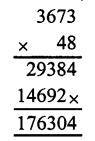(v) 4.125 x 86=354.750 (Three places of decimal)
= 354.75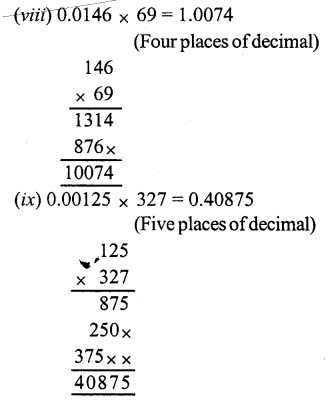Question 5.
Solution:
(i) 7.6 x 2.4= 18.24
{Sum of decimal places = 1 + 1 = 2}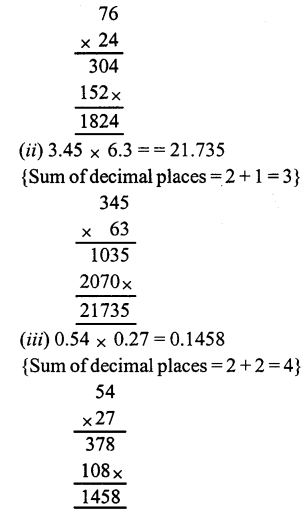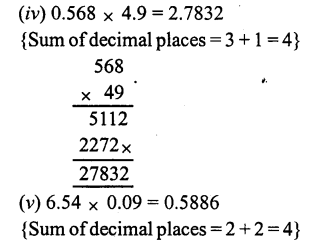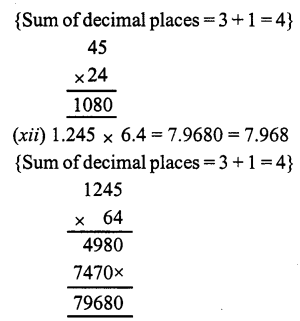Question 6.
Solution:
(i) 13 x 1.3 x 0.13 = 2.197
{Sum of decimal places = 1 + 2 = 3}(ii) 2.4 x 1.5 x 2.5 = 9.000 = 9
{Sum of decimal places = 1 + 1 + 1 = 3}
(iii) 0.8 x 3.5 x 0.05 = 0.1400 = 0.14
{Sum of decimal places = 1 + 1 + 2 = 4}
(iv) 0.2 x 0.02 x 0.002 = 0.000008
{Sum of decimal places = 1 + 2 + 3 = 6}
(v) 11.1 x 1.1 x 0.11 = 1.3431
{Sum of decimal places = 1 + 1 + 2 = 4}(vi) 2.1 x 0.21 x 0.021 = 0.00926
21 x 21 = 441
441 x 21 = 9261
{Sum of decimal places = 1 + 2 + 3 = 6}

Question 7.
Solution:
(i) (1.2)²= 1.2 x 1.2 = 1.44
{Sum of decimal places = 1 + 1 = 2}
(ii) (0.7)² = 0.7 x 0.7 = 0.49
{Sum of decimal places = 1 + 1 = 2}
(iii) (0.04)² = 0.04 x 0.04 = 0.0016
{Sum of decimal places = 2 + 2 = 4}
(iv) (0.11)² = 0.11 x 0.11 =0.0121
{Sum of decimal places = 2 + 2 = 4}

Question 8.
Solution:
(i) (0.3)3 = 0.3 x 0.3 x 0.3 = 0.027
{Sum of decimal places = 1 + 1 + 1 = 3}
(ii) (0.05)3= 0.05 x 0.05 x 0.05 = 0.000125
{Sum of decimal places = 2 + 2 + 2 = 6}
(iii) (1.5)3 = 1.5 x 1.5 x 1.5 = 3.375
{Sum of decimal places = 1 + 1 + 1 = 3}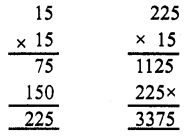Question 9.
Solution:
Distance covered in one hour = 62.5 km
Distance covered in 18 hours = 62.5 x 18 km = 1125.0 km

Question 10.
Solution:
Weight of one tin of oil = 16.8 kg
Weight of 45 tins = 16.8 x 45 kg = 756.0 kg = 756 kgQuestion 11.
Solution:
Weight of wheat in one bag = 97.8 kg
weight of wheat in 500 bags = 97.8 x 500 kg = 48900.0 kg = 48900 kgQuestion 12.
Solution:
Weight of one bag = 48.450 kg
Weight of 16 bags = 48.450 x 16 = 775.200 kg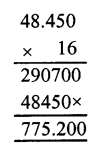Question 13.
Solution:
Quantity of sauce in one bottle = 0.845 kg
quantity of sauce in 72 bottles = 0.845 x 72 kg = 60.840 kgQuestion 14.
Solution:
Quantity of jam in one bottle = 925 .
Quantity of jam in 25 bottles = 925 x 25 g = 23135 g = 23.125 kg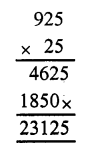Question 15.
Solution:
Oil in one drum = 16.850 litres
Oil in 48 drums = 16.850 x 48 = 808.800 = 808.800 litresQuestion 16.
Solution:
Cost of 1 kg rice = Rs 56.80
Cost of 16.25 kg of rice = Rs 56.80 x 16.25 = Rs 923.0000 = Rs 923Question 17.
Solution:
Cost of one metre of cloth = Rs 108.5 0
Costof 18.5 metres of cloth = Rs 108.50 x 18.5 = Rs 2007.250 = Rs 2007.25Question 18.
Solution:
Distance covered in one litre = 8.6 km
Distance covered in 36.5 litres = 8.6 x 36.5 km = 313.90 km = 313.9 kmQuestion 19.
Solution:
Charges for 1 km = Rs 9.80
Charges for 106.5 km = Rs 9.80 x 106.5 = Rs 1043.700 = Rs 1043.70All Chapter RS Aggarwal Solutions For Class 7 Maths

—————————————————————————–

All Subject NCERT Exemplar Problems Solutions For Class 7

All Subject NCERT Solutions For Class 7

*************************************************

I think you got complete solutions for this chapter. If You have any queries regarding this chapter, please comment on the below section our subject teacher will answer you. We tried our best to give complete solutions so you got good marks in your exam.

If these solutions have helped you, you can also share rsaggarwalsolutions.in to your friends.